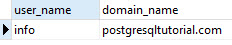# PostgreSQL Tutorial: LENGTH Function

August 1, 2023

Summary: in this tutorial, we will show you various PostgreSQL length functions that return the number of characters or the number of bytes of a string.

## PostgreSQL LENGTH function

The following illustrates the syntax of the length function:

``````LENGTH(string);
``````

The length function accepts a string as a parameter. A string can be any of the following data types:

• character or char
• character varying or varchar
• text

The length function returns the number of characters in the string.

### PostgreSQL length function examples

See the following example of using the length function:

``````SELECT
LENGTH ('PostgreSQL Tutorial'); -- 19
``````

Notice that a string can hold an empty string, which is not a null value.

``````SELECT
LENGTH (''); -- 0
``````

It returns zero. However, a string that holds a space character:

``````SELECT
LENGTH (' '); -- 1
``````

It returns 1.

If you pass a NULL value to the length function, it returns a NULL value.

``````SELECT
LENGTH (NULL); -- NULL
``````

The following query gets the full names of customers in the `customer` table and uses the length function to get the number of characters in their names.

``````SELECT
first_name || ' ' || last_name AS name,
LENGTH (first_name || ' ' || last_name) len
FROM
customer
ORDER BY
len;
``````Sometimes, you may want to measure the length of a number instead of a string. In this case, you use type cast to convert the number into a string and use the length function as the following example:

``````SELECT
LENGTH (CAST(12345 AS TEXT)); --- 5
``````

We often use the length function with other string functions such as replace, substring, etc., to manipulate string more efficiently. The following statement gets the user name and domain from the an email address using `substring`, `strpos`, and `length`functions.

``````SELECT
SUBSTRING (
'info@rockdata.net',
1,
strpos(
'info@rockdata.net',
'@'
) - 1
) AS user_name,
SUBSTRING (
'info@rockdata.net',
strpos(
'info@rockdata.net',
'@'
) + 1,
LENGTH (
'info@rockdata.net'
)
) AS domain_name;
``````Besides the length function, PostgreSQL provides the `char_length` and `character_length` functions that provide the same functionality.

## Measure strings in bytes and bits

To get the number of bytes in a string, you use the octet_length function as follows:

``````OCTET_LENGTH(string);
``````

See the following example:

``````SELECT
OCTET_LENGTH ('A'); -- 1 byte
``````

It returns 1 byte.

``````SELECT
OCTET_LENGTH ('€'); -- 3 bytes
``````

It returns 3 bytes. However, with the length function, it returns just 1.

``````SELECT
LENGTH ('€'); -- 1
``````

To measure the number of bits of a string, you use the `bit_length` function as follows:

``````BIT_LENGTH(string);
``````

See the following examples of using the `bit_length` function.

``````SELECT
BIT_LENGTH ('A'); -- 8 bits
``````
``````SELECT
BIT_LENGTH ('€'); -- 24 bits
``````

In this tutorial, we have shown you how to use length, bit_length, and octet_length functions to return the number of characters, the number of bits, and the number of bytes of a string.